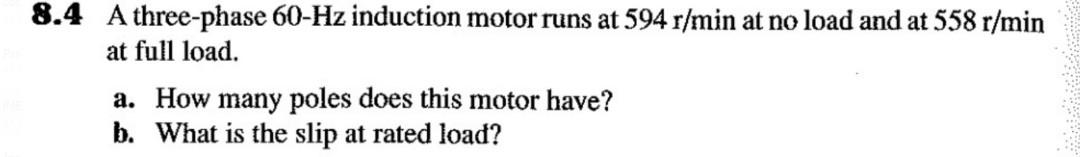# Question 8.4 A three-phase 60-Hz induction motor runs at 594 r/min at no load and at 558 r/min at full load. a. How many poles does this motor have? b. What is the slip at rated load? 8.4 A three-phase 60-Hz induction motor runs at 594 r/min at no load and at 558 r/min at full load. a. How many poles does this motor have? b. What is the slip at rated load?1LSQWJ The Asker · Electrical EngineeringTranscribed Image Text: 8.4 A three-phase 60-Hz induction motor runs at 594 r/min at no load and at 558 r/min at full load. a. How many poles does this motor have? b. What is the slip at rated load?
More
Transcribed Image Text: 8.4 A three-phase 60-Hz induction motor runs at 594 r/min at no load and at 558 r/min at full load. a. How many poles does this motor have? b. What is the slip at rated load?